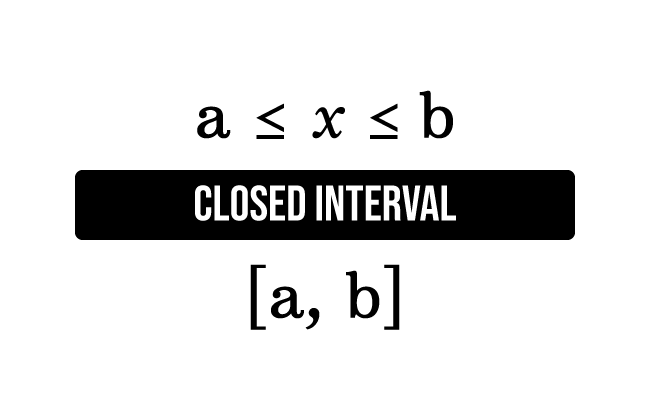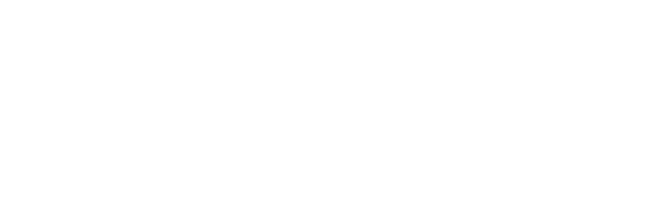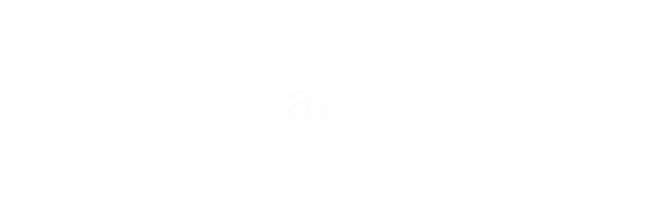# Closed interval

An interval that represents a set of members by including both lower and higher values is called a closed interval.

## Introduction

According to the Set Theory, the members (or elements) are collected to represent their collection as a set. Actually, all elements lie between two members. Hence, all members can be expressed as an interval of two members. In this case, a set should be expressed by including the lower and higher value members and it is called a closed interval.$x \ge a$ and $x \le b$

The two inequalities tell the following two factors in mathematical form.

1. The value of $x$ is greater than or equal to $a$.
2. The value of $x$ is less than or equal to $b$.

For our convenience, the two mathematical statements can also be written as follows.

$a \le x \le b$

This single mathematical inequality expresses that the value of $x$ lies between $a$ and $b$, and also equals to $a$ and $b$. Hence, this mathematical inequality is written as a closed interval between $a$ and $b$.

### Representation

In mathematics, a closed interval is represented in two different ways.

#### Graphical Representation

A closed interval is represented in graphical system by considering the following two factors.1. An interval is graphically represented by a number line.
2. The endpoints of the number-line are represented by the filled circles (or darkened circles) for saying that the lower and higher values are also considered.

#### Mathematical Representation

A closed interval is represented in mathematical form by considering the following two factors.1. The lower and higher quantities are written in a row and they are separated by a comma $(,)$ and some space between them.
2. A pair of square brackets are written before and after the lower and higher value elements to tell that their values are also included.

Therefore, a closed interval between $a$ and $b$ is written as $x \,∈\, \big[a, b\big]$

As per the set builder notation, a closed interval between $a$ and $b$ is written in the following forms.

$(1).\,\,\,$ $\Big\{x \,\,|\,\, x \,∈\, R \,\, and \,\, a \, \le \, x \, \le \, b\Big\}$

$(2).\,\,\,$ $\Big\{x \,:\, x \,∈\, R \,\, and \,\, a \, \le \, x \, \le \, b\Big\}$

##### Example

Evaluate $f(x)$ if $f(x) = x+1$ where $x \,∈\, \big[2, 5\big]$

Let’s understand the concept of closed interval from this simple example. In this problem, the value of the function has to evaluate for every value of $x$ but the value of $x$ should be from $2$ to $5$. So, find the value of function $f(x)$ by substituting the value of $x$ from $2$ to $5$.

$f(2) \,=\, 2+1 = 3$

$f(3) \,=\, 3+1 = 4$

$f(4) \,=\, 4+1 = 5$

$f(5) \,=\, 5+1 = 6$

Latest Math Topics
Jun 26, 2023
Jun 23, 2023

###### Math Questions

The math problems with solutions to learn how to solve a problem.

Learn solutions

Practice now

###### Math Videos

The math videos tutorials with visual graphics to learn every concept.

Watch now

###### Subscribe us

Get the latest math updates from the Math Doubts by subscribing us.